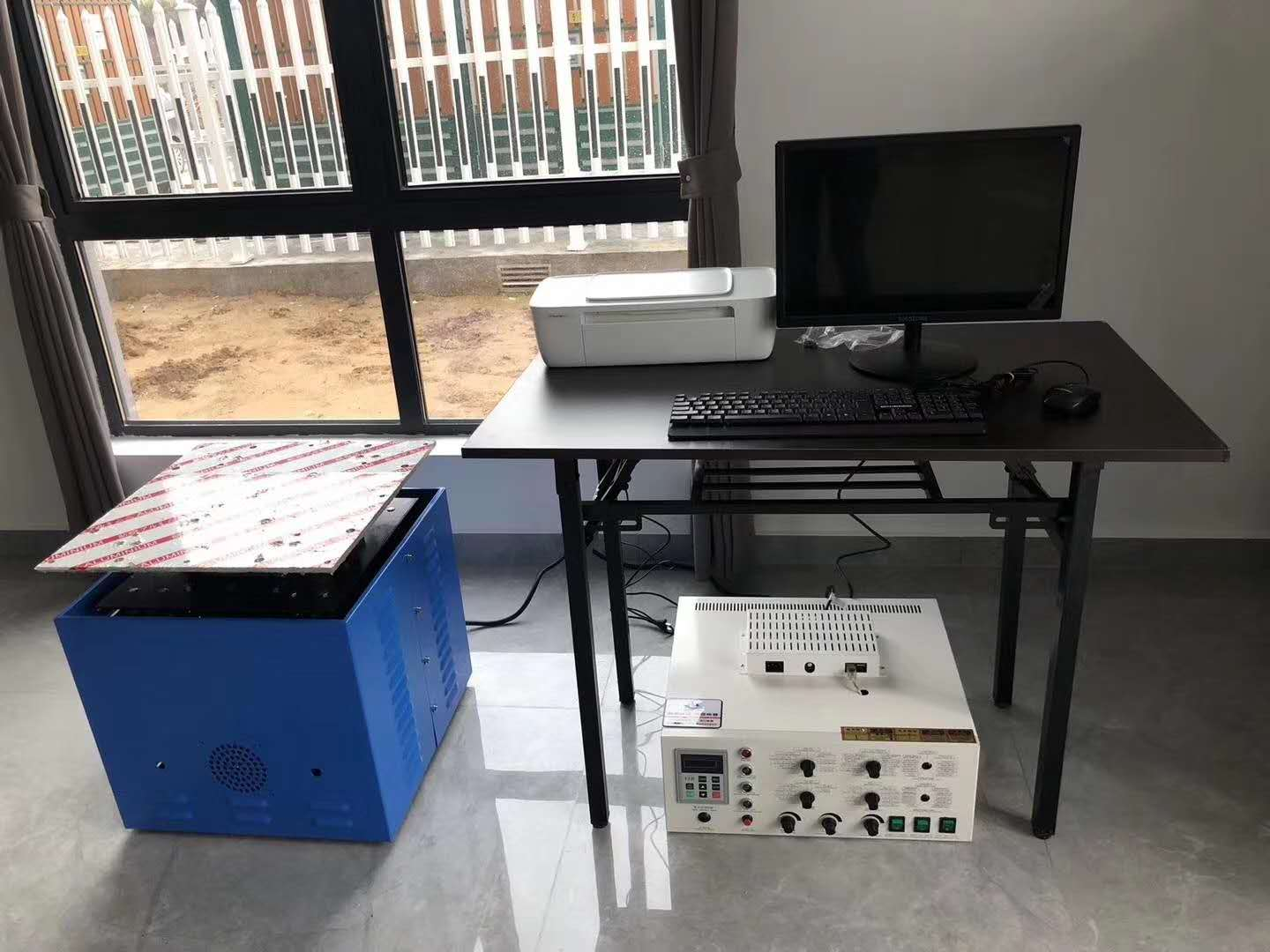18925802250
Home>>新闻资讯

# 分享振动台单组操作方法

### 发布日期：2022年07月27日浏览量：62

1.单组操作: 先按总复位开关,面板显示C00，调CD065=0，CD041=1 ①CD012=CD013=0.1 ②CD000= 所要设定频率 (1-600HZ) ③CD087= 执行时间 (0-65500秒) ④CD064为CD087的运转次数,总时间=CD087(秒)×CD064(次) ⑤CD020到CD027=0,CD080到CD086=0,CD088到CD094=0, CD098到CD105=0 ⑥调CD003与CD005为调幅开关(CD003>CD005 ,两者数字越大则振动相对越大,反之则小,视设定频率大小而决定) ⑦面板选择开关<启动开关>打到垂直<上下>面板即可运行当前设定参数 ⑧可在HZ灯亮(『00.00画面状态）看现在运行的频率. ⑨ .(STOP/RESET) 为停止开关 ⑩当设定时间结束后,机器会自动停止,按总复位开关显示C00即可重新运行

.单组操作: 例2:10HZ.4小时振幅2mm,如下设定： 例4:100HZ.4小时加速度10G如下设定 ①按总复位开关，面板显示C00即松开 ①按总复位开关，面板显示C00即松开 ②调CD065=0，CD041=1<如默认正确则不用设定> ②调CD065=0，CD041=1<如默认正确则不用设定> ③CD012=0.1<固定程序> ③CD012=0.1<固定程序>④CD013=0.1<固定程序> ④CD013=0.1<固定程序> ⑤CD000=10 <即所要设定的频率> ⑤CD000=100 <即所要设定的频率> ⑥CD087=3600 <秒为单位,即1小时> ⑥CD087=3600 <秒为单位,即1小时> ⑦CD064=4<运转次数,即总时间=CD087*CD064=4小时> ⑦CD064=4<运转次数,即总时间=CD087*CD064=4小时> ⑧CD020到CD027=0 ⑧CD020到CD027=0 CD080到CD086=0 CD080到CD086=0 CD088到CD094=0 CD088到CD094=0 CD094到CD105=0 CD094到CD105=0 ⑨CD003=68 <振幅设定>（可参考振幅参考值） ⑨CD003=65 <振幅设定>（可参考振幅参考值） ⑩CD005=25 <振幅设定>（可参考振幅参考值） ⑩CD005=20 <振幅设定>（可参考振幅参考值） ⑾面板选择开关<启动开关>打到垂直<上下>面板即可运行 ⑾面板选择开关<启动开关>打到垂直<上下>面板即可运行 当前设定参数 当前设定参数 ⑿在HZ灯亮,『00.00画面状态可看到运行频率 ⑿在HZ灯亮,『00.00画面状态可看到运行频率 ⒀按 STOP 键为停止 ⒀按 STOP 键为停止 RESET RESET ⒁当设定的时间结束后,机器会自动停止,按总复位开关显示 ⒁当设定的时间结束后,机器会自动停止,按总复位开关显示 C00即可重新运行 C00即可重新运行Top